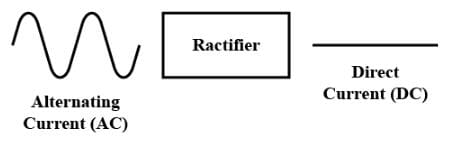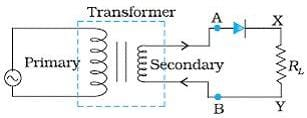NEET  >  Test: Applications of P-N Diode

# Test: Applications of P-N Diode

Test Description

## 10 Questions MCQ Test Physics Class 12 | Test: Applications of P-N Diode

Test: Applications of P-N Diode for NEET 2023 is part of Physics Class 12 preparation. The Test: Applications of P-N Diode questions and answers have been prepared according to the NEET exam syllabus.The Test: Applications of P-N Diode MCQs are made for NEET 2023 Exam. Find important definitions, questions, notes, meanings, examples, exercises, MCQs and online tests for Test: Applications of P-N Diode below.
Solutions of Test: Applications of P-N Diode questions in English are available as part of our Physics Class 12 for NEET & Test: Applications of P-N Diode solutions in Hindi for Physics Class 12 course. Download more important topics, notes, lectures and mock test series for NEET Exam by signing up for free. Attempt Test: Applications of P-N Diode | 10 questions in 10 minutes | Mock test for NEET preparation | Free important questions MCQ to study Physics Class 12 for NEET Exam | Download free PDF with solutions
 1 Crore+ students have signed up on EduRev. Have you?
Test: Applications of P-N Diode - Question 1

### The knee voltage of a-n junction diode is 0.8 V and the with of the depletion layer is 2 μm. What is the electric field in the depletion layer?​

Detailed Solution for Test: Applications of P-N Diode - Question 1

Knee voltage- it is that forward voltage beyond which current start increasing rapidly, but below knee voltage variation of forward current and applied voltage is linear.
The electric field in a region is given by, E=V/l.
where V is the potential and l is the length or distance of the region in which it has to be measured.
now, E=V/l
E=0.8/2×10-6 m
E= 0.4 MV/m

Test: Applications of P-N Diode - Question 2

### An electronic device which converts a.c power into d.c power is

Detailed Solution for Test: Applications of P-N Diode - Question 2

A rectifier is a device which converts a.c (alternating current) to d.c( direct current), which flows in only one direction. This process is known as Rectification.
This is done using a p-n junction diode.
A p-n junction diode allows electric current in only forward bias condition and blocks electric current in reverse bias conditions.
In other words, a diode allows electric current in one direction. This unique property of diode allows it to act like a Rectifier.Test: Applications of P-N Diode - Question 3

### In a half wave rectifier, the secondary coil S of the transformer is connected to​

Detailed Solution for Test: Applications of P-N Diode - Question 3In a half wave rectifier, the secondary coil S of the transformer is connected to​ both junction diode and a load resistance.

Test: Applications of P-N Diode - Question 4

A 50 Hz a.c. is rectified with full wave rectifier, what is the output frequency?​

Detailed Solution for Test: Applications of P-N Diode - Question 4

In full wave rectification the input frequency is doubled. Because all the negative components in the AC input signal are converted into positive components. Hence, the positive components are doubled.
Therefore in full wave rectification output frequency will be 100 Hz i.e. double that of input frequency 50 Hz.

Test: Applications of P-N Diode - Question 5

The conductivity of a photosensitive semiconductor

Detailed Solution for Test: Applications of P-N Diode - Question 5

Photoconductivity, as a well-known optical and electrical phenomenon in semiconductor, is an effect that the electrical conductivity increases due to the absorption of light radiation.

Test: Applications of P-N Diode - Question 6

Zener diodes are used as

Detailed Solution for Test: Applications of P-N Diode - Question 6

Zener Diodes can be used to produce a stabilised voltage output with low ripple under varying load current conditions. By passing a small current through the diode from a voltage source, via a suitable current limiting resistor (RS), the zener diode will conduct sufficient current to maintain a voltage drop of Vout.

Test: Applications of P-N Diode - Question 7

The current that exists in the circuit of a photodiode even when no visible light is made incident on it is called

Detailed Solution for Test: Applications of P-N Diode - Question 7

In physics and in electronic engineering, dark current is the relatively small electric current that flows through photosensitive devices such as a photomultiplier tube, photodiode, or charge-coupled device even when no photons are entering the device; it consists of the charges generated in the detector when no outside radiation is entering the detector. It is referred to as reverse bias leakage current in non-optical devices and is present in all diodes. Physically, dark current is due to the random generation of electrons and holes within the depletion region of the device.

Test: Applications of P-N Diode - Question 8

The efficiency of a full wave rectifier is

Detailed Solution for Test: Applications of P-N Diode - Question 8

Efficiency of half-wave and full wave rectifier is given by
ηh​=40.6% and ηf​=81.2%
So,  ηhf​​​=40.6/81.2 ​=1/2​
∴f/n ​=2ηh​
Hence, the efficiency of a full wave rectifier is double of half wave-rectifier.

Test: Applications of P-N Diode - Question 9

The energy of radiation emitted by LED is

Detailed Solution for Test: Applications of P-N Diode - Question 9

This energy is emitted in the form of heat and light. The electrons dissipate energy in the form of heat for silicon and germanium diodes but in gallium arsenide phosphide (GaAsP) and gallium phosphide (GaP) semiconductors, the electrons dissipate energy by emitting photons.

Test: Applications of P-N Diode - Question 10

A diode as a rectifier converts:​

Detailed Solution for Test: Applications of P-N Diode - Question 10

A diode as a rectifier is used for converting AC to DC.
Following are the applications of the diode as a rectifier:
It is used for the mixing of signals.
It is used for the detection of signals.
Used in lighting systems.
When a diode is used as a rectifier, it acts as a two-lead semiconductor which allows current to pass from only one direction.

## Physics Class 12

157 videos|452 docs|213 tests
 Use Code STAYHOME200 and get INR 200 additional OFF Use Coupon Code
Information about Test: Applications of P-N Diode Page
In this test you can find the Exam questions for Test: Applications of P-N Diode solved & explained in the simplest way possible. Besides giving Questions and answers for Test: Applications of P-N Diode, EduRev gives you an ample number of Online tests for practice

## Physics Class 12

157 videos|452 docs|213 tests

### How to Prepare for NEET

Read our guide to prepare for NEET which is created by Toppers & the best Teachers